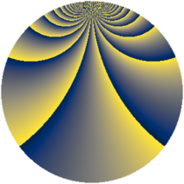# Properties

 Label 2240.2.bjLevel $2240$ Weight $2$ Character orbit 2240.bj Rep. character $\chi_{2240}(1217,\cdot)$ Character field $\Q(\zeta_{4})$ Dimension $184$ Sturm bound $768$

# Related objects

## Defining parameters

 Level: $$N$$ $$=$$ $$2240 = 2^{6} \cdot 5 \cdot 7$$ Weight: $$k$$ $$=$$ $$2$$ Character orbit: $$[\chi]$$ $$=$$ 2240.bj (of order $$4$$ and degree $$2$$) Character conductor: $$\operatorname{cond}(\chi)$$ $$=$$ $$35$$ Character field: $$\Q(i)$$ Sturm bound: $$768$$

## Dimensions

The following table gives the dimensions of various subspaces of $$M_{2}(2240, [\chi])$$.

Total New Old
Modular forms 816 200 616
Cusp forms 720 184 536
Eisenstein series 96 16 80

## Trace form

 $$184q + O(q^{10})$$ $$184q + 8q^{21} - 8q^{25} + 8q^{37} + 8q^{53} - 32q^{57} - 8q^{65} + 80q^{77} - 200q^{81} - 88q^{85} - 16q^{93} + O(q^{100})$$

## Decomposition of $$S_{2}^{\mathrm{new}}(2240, [\chi])$$ into newform subspaces

The newforms in this space have not yet been added to the LMFDB.

## Decomposition of $$S_{2}^{\mathrm{old}}(2240, [\chi])$$ into lower level spaces

$$S_{2}^{\mathrm{old}}(2240, [\chi]) \cong$$ $$S_{2}^{\mathrm{new}}(35, [\chi])$$$$^{\oplus 7}$$$$\oplus$$$$S_{2}^{\mathrm{new}}(70, [\chi])$$$$^{\oplus 6}$$$$\oplus$$$$S_{2}^{\mathrm{new}}(140, [\chi])$$$$^{\oplus 5}$$$$\oplus$$$$S_{2}^{\mathrm{new}}(280, [\chi])$$$$^{\oplus 4}$$$$\oplus$$$$S_{2}^{\mathrm{new}}(560, [\chi])$$$$^{\oplus 3}$$$$\oplus$$$$S_{2}^{\mathrm{new}}(1120, [\chi])$$$$^{\oplus 2}$$